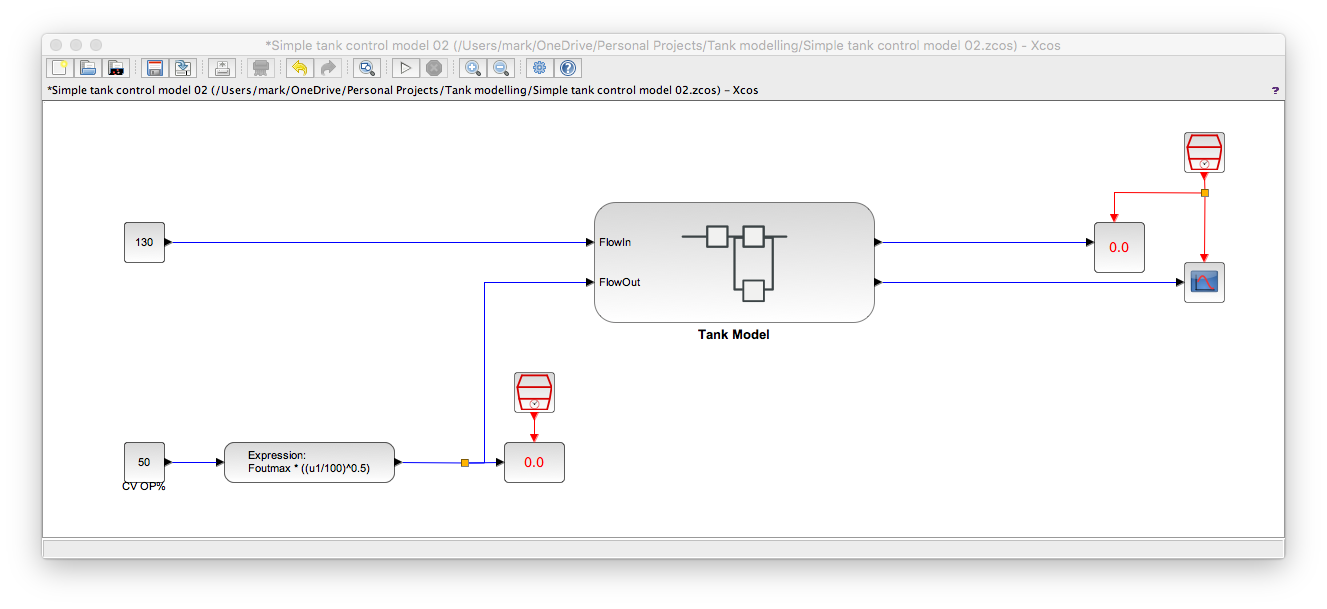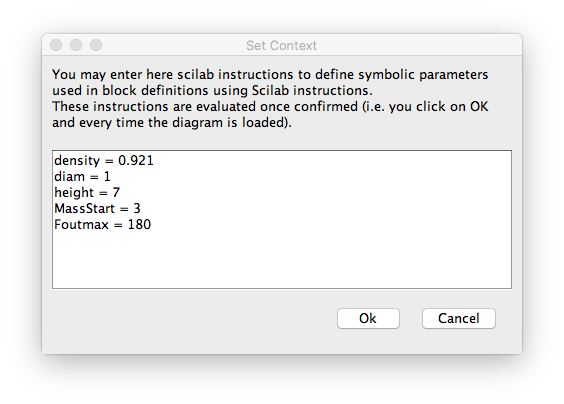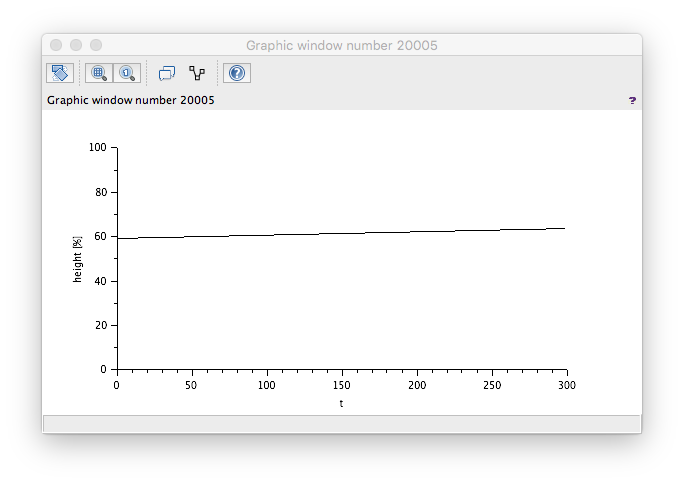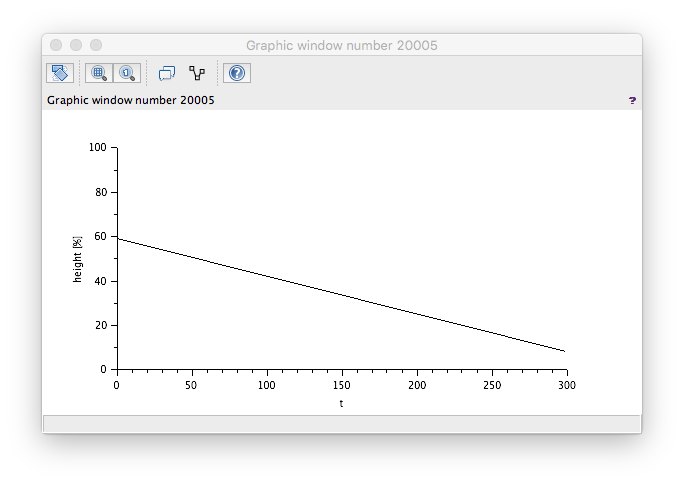Simple tank model development - Part 2

Adding a control valve to the tank model.

[This is part 2: part 1 builds a tank model, part 3 adds a controller.]

Taking the tank model developed previously we can add a model for the control valve.The tank model sketch showed that the control valve had a square-root trim hence the expression used in the above. Foutmax is the maximum throughput of the valve.

Note that the above ignores differential pressure! But this is OK because we are using my principle of real enough modelling and we are not so intersted in a high-fidelity valve model. We want to be able to answer the question, "while my proposed control philosophy work in principle?".

The context for the above is...If we run this model with settings of flow in = 130 and CV % = 50% we get ...which is as expected since 50% position will give 127.3 Te/hr flow so we should be slowly filling the tank.

If we re-run but have CV% = 80% we get...which again is as expected since 80% position is 161.0 Te/hr flow so we should be emptying the tank quickly.

The Xcos model for the above can be downloaded via...[This is part 2: part 1 builds a tank model, part 3 adds a controller.